Get instant live expert help with Excel or Google Sheets“My Excelchat expert helped me in less than 20 minutes, saving me what would have been 5 hours of work!”

#### Post your problem and you’ll get Expert help in seconds.

Your message must be at least 40 characters
Our professional Expert are available now. Your privacy is guaranteed.

# A Guide to Using Conditional Data Validation

Data Validation is an Excel feature whose purpose is to restrict what users can input into a cell. It is essentially for creating drop-down lists or combo boxes that contain predefined options that limit user errors and allow for more consistent data entry.

Sometimes, however, we may want to use more than one drop-down list in such a way that the options included in a second drop-down list are dependent on the choices made in the first drop-down list. This is what we call conditional data validation.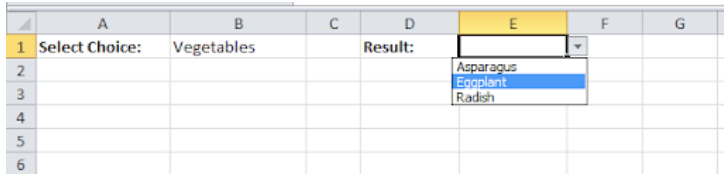Figure 1. How to use conditional data validation

## What is Conditional Data Validation?

Conditional data validation means restricting the choices in an Excel drop-down list depending on the value in another cell (or in another drop down, for that matter). Here, we will find out how to create conditional Excel drop down using Data Validation.

## Steps in Using Conditional Data Validation

Here is what we are attempting to accomplish in this example. The user selects Fruits from a drop-down list.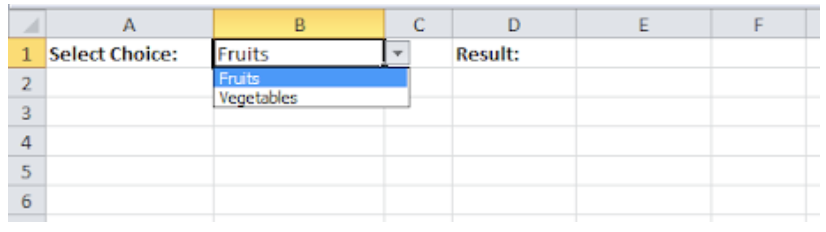Figure 2. How to use conditional data validation

As a result, the second drop-down list contains the Fruits items.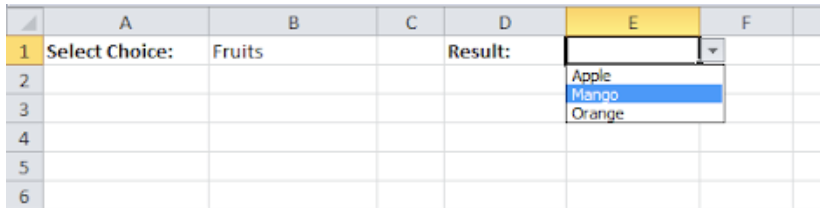Figure 3. How to use conditional data validation

The second drop-down list is dependent on the value selected from the first drop-down list. To create these dependent drop-down lists, we will perform the following steps:

• Step 1. On the second sheet, create the named ranges shown in the table below. A named range is a range (a group of cells in a worksheet that are highlighted or selected) that has been assigned a specific and/or descriptive name. It is important to name the ranges that we are going to use in order to make it easier to reference them.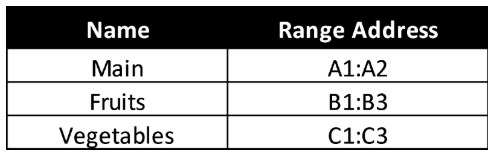Figure 4. How to use conditional data validation

One way to name a range is by selecting the group of cells we want to use, then clicking the Name Box located at the left of the formula bar, typing in the desired one-word name, and pressing the Enter key.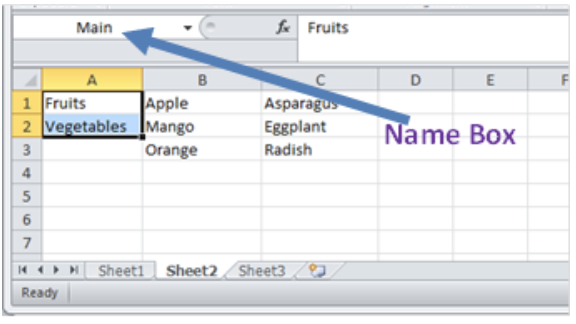Figure 5. How to use conditional data validation

• Step 2. On the first sheet, select cell B1.
• Step 3. On the Data tab, in the Data Tools group, click Data Validation.Figure 6. How to use conditional data validation

The Data Validation dialog box appears.

• Step 4. In the Allow box, click List.
• Step 5. Click in the Source box and type =Main.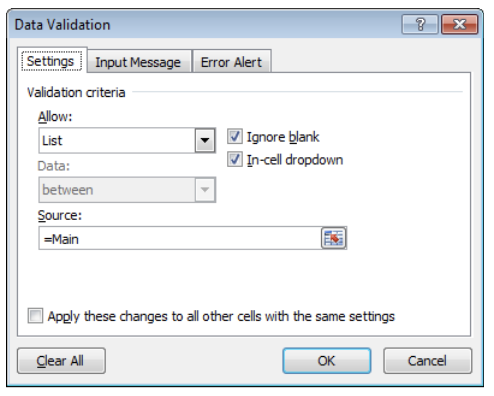Figure 7. How to use conditional data validation

• Step 6. Click OK.

This will result in the creation of the choices for the main drop down.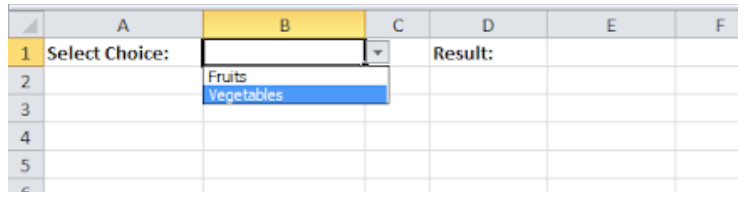Figure 8. How to use conditional data validation

• Step 7. The next step is to select cell E1.
• Step 8. In the Allow box, click List.
• Step 9. Click in the Source box and type =INDIRECT(\$B\$1).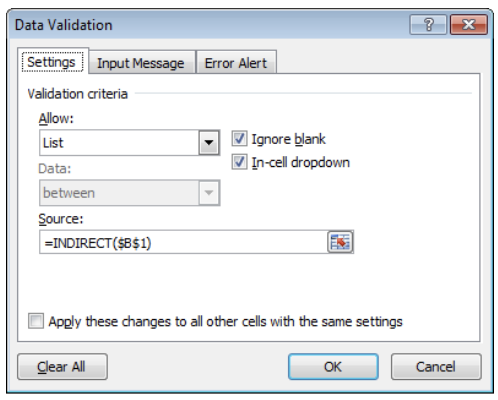Figure 9. How to use conditional data validation

• Step 10. Click OK.

This will result in the creation of the choices for the second drop-down list.Figure 10. How to use conditional data validation

## The INDIRECT Function

The INDIRECT function returns a reference to a range. In this formula, INDIRECT simply evaluates values in column B as references, which links them to the named ranges we have defined previously. For example, if the user chooses Vegetables from the first drop-down list, the function INDIRECT(\$B\$1) returns the Vegetable reference. The second drop-down list will then show the Vegetable items. We can check the following link for more details on the INDIRECT function:

https://www.got-it.ai/solutions/excel-chat/excel-tutorial/lookup-and-reference/excel-indirect-function

Most of the time, the problem you will need to solve will be more complex than a simple application of a formula or function. If you want to save hours of research and frustration, try our live Excelchat service! Our Excel Experts are available 24/7 to answer any Excel question you may have. We guarantee a connection within 30 seconds and a customized solution within 20 minutes.

Are you still looking for help with Conditional Formatting? View our comprehensive round-up of Conditional Formatting tutorials here.

### Did this post not answer your question? Get a solution from connecting with the expert.Another blog reader asked this question today on Excelchat:
Solution examplesI have a google sheet that takes information from a google form, that creates a row every time a form is submitted. Since it is a new row that is created, rather than filling in the next row down, the formulas I have applied to some of the columns do not work. The same is true when I try and link the data to another sheet to bypass this problem. This is probably quite a general functionality issue so I won't send the actual document but let me know if you need it
Solved by F. F. in 13 minsHello, I need help regarding indirect formula of excel. 1. What is the purpose of using this formula? 2. If we use this formula with sum formula, then why we are using this with sum formula, we can also perform addition from different sheets without indirect formula ?
Solved by O. D. in 21 minsI want to dynamic access a sheet/cell from a closed file using INDEX. But when I try to "&A3&" for sheet name I forces me to select a file and then populates the sheet name instead of making it dynamically INDEX('/Users/me/Desktop/["&A2&"]"&A3&"'!A:A,1,1)
Solved by G. H. in 20 minsI need explanation for the excel formula below... =IF(ISERROR(SUMIFS(INDIRECT("'"&BE\$6&"'!"&"\$G:\$G"),INDIRECT("'"&BE\$6&"'!"&"\$R:\$R"),\$A39,INDIRECT("'"&BE\$6&"'!"&"\$F:\$F"),"<>52711",INDIRECT("'"&BE\$6&"'!"&"\$C:\$C"),10)),0,SUMIFS(INDIRECT("'"&BE\$6&"'!"&"\$G:\$G"),INDIRECT("'"&BE\$6&"'!"&"\$R:\$R"),\$A39,INDIRECT("'"&BE\$6&"'!"&"\$F:\$F"),"<>52711",INDIRECT("'"&BE\$6&"'!"&"\$C:\$C"),10))+IF(ISERROR(SUMIFS(INDIRECT("'"&BE\$4&"'!"&"\$A\$30:\$EO\$30"),INDIRECT("'"&BE\$4&"'!"&"\$A\$4:\$EO\$4"),BE\$5,INDIRECT("'"&BE\$4&"'!"&"\$A\$5:\$EO\$5"),\$A39)),0,SUMIFS(INDIRECT("'"&BE\$4&"'!"&"\$A\$30:\$EO\$30"),INDIRECT("'"&BE\$4&"'!"&"\$A\$4:\$EO\$4"),BE\$5,INDIRECT("'"&BE\$4&"'!"&"\$A\$5:\$EO\$5"),\$A39))+IF(ISERROR(SUMIF(INDIRECT("'"&BE\$6&" "&\$A\$31&"'!"&"H:H"),\$A39,(INDIRECT("'"&BE\$6&" "&\$A\$31&"'!"&"I:I")))),0,SUMIF(INDIRECT("'"&BE\$6&" "&\$A\$31&"'!"&"H:H"),\$A39,(INDIRECT("'"&BE\$6&" "&\$A\$31&"'!"&"I:I"))))
Solved by V. A. in 20 mins## Subscribe to Excelchat.coAnother blog reader asked this question today on Excelchat: